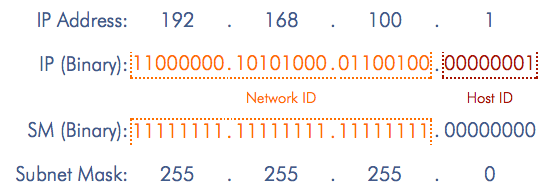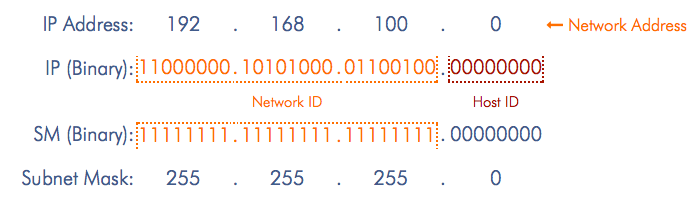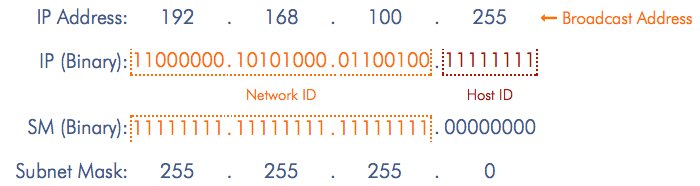BLOG HOME

# Techniques to master IP Subnetting - Part 1Let's start with the "why" first. Why should we put in the effort to master subnetting? The answer is simple - it is essential to master subnetting if one wants to pursue a career in computer networking. Why are we writing a post on the topic? Our purpose is simply to demonstrate that subnetting is not as challenging a topic as it might sometimes seem.

The first step in simplifying everything related to subnetting is to identify the key outcomes we are aiming for:

• Break up a range of IP Addresses into a number of smaller ranges (subnets)
• Summarize a number of contiguous subnets into a single network

### Identifying network boundaries

Of the three outcomes we outlined above, identifying network boundaries is the most important one. This forms the basis of designing IP-based routed networks. This post will focus exclusively on this outcome. Once we master this outcome, the other 2 outcomes mentioned above become much simpler to master.

We can define our requirement as follows:

### Pro Tip #1: Understand binary numbers - but don't get hung up on them

Each digit of a binary number can one of 2 values - 0 or 1. Each digit is referred to as a bit. The same number (for example the number 123) requires many more digits to represent in binary (1111011 - 7 digits) than in decimal (123 - 3 digits).

8 binary digits (bits) can represent 256 different values (the decimal numbers 0 to 255). We use 8-bit blocks to store data in digital form. A block of 8 bits is often referred to as a byte - but more correctly as an 'octet'.

Here is an example with the IP Address 192.168.0.1, Subnet Mask 255.255.255.0. The first 24 bits of the IP Address represent the Network ID, the remaining 8 bits represent the Host ID.The Network Address is 192.168.100.0 - all of the Host ID bits are set to 0:Network Address: 192.168.0.0 - All of the Host ID bits are set to 0

The Broadcast Address is 192.168.100.255 - all of the Host ID bits are set to 1:Broadcast Address: 192.168.0.255 - All of the Host ID bits are set to 1

Does this mean that we need to convert a Decimal IP Address and Subnet Mask into Binary every time we want to figure out the network and broadcast address? Fortunately, the answer is no! We can quickly figure out the Network Address and Broadcast Address given any IP Address and Subnet Mask without ever needing to convert anything into binary!

### Pro Tip #2: Recognize the 9 possible values in any octet of a Subnet Mask

Subnet Masks range from 0.0.0.0 to 255.255.255.255 - however, a Subnet Mask cannnot have any random value within this range. Each octet of a Subnet Mask can have one of only 9 possible values:

 0 128 192 224 240 248 252 254 255

A detailed explanation of IP Addresses and Subnet Masks is covered here.

### Terminology - Interesting Octet

While reading up on Subnetting, you may have come across the term "Interesting Octet". For the purposes of this post, we will define the term as follows:

Given an IP Address and Subnet Mask, the "Interesting Octet" is the first octet of the Subnet Mask whose value is not 255.

For example, given the Subnet Mask 255.255.248.0, the Interesting Octet is Octet 3.

### Pro Tip #3: Become very familiar with the powers of 2 (and their multiples)

The key to becoming adept at subnetting is to become really familiar with the powers of 2 (up to 28). You should be able to easily recognize the following numbers:

 0 2 4 8 16 32 64 128 256

Subtracting these values from 256, you should be able to quickly recognize the following values:

 256 256 256 256 256 256 256 256 -2 -4 -8 -16 -32 -64 -128 -256 254 252 248 240 224 192 128 0

These values above are values found in the Interesting Octet of a Subnet Mask. What is the relationship between the Interesting Octet of a Subnet Mask and the Interesting Octet of the corresponding Network Address?

The Interesting Octet of the Network Address will be a multiple of 256 - interesting octet of the Subnet Mask as the following table shows:

 256 256 256 256 256 256 256 256 -2 -4 -8 -16 -32 -64 -128 -256 Interesting Octetof Subnet Mask: 254 252 248 240 224 192 128 0 Interesting Octet ofNetwork Address: multiple of 2 multiple of 4 multiple of 8 multiple of 16 multiple of 32 multiple of 64 multiple of 128 0 Interesting Octet ofNetwork Address:

### Pro Tip #4: Understand and remember the table above - the relationship between the Interesting Octet of a Subnet Mask, and the Interesting Octet of the corresponding Network Address

For example, if the Interesting Octet of the Subnet Mask is 128, the corresponding Interesting Octet of the Network Address will be one of the following (multiples of 128 that are less than 256):

 Interesting Octet of Subnet Mask: 128 Interesting Octet ofNetwork Address: 0 128

If the Interesting Octet of the Subnet Mask is 192, the corresponding Interesting Octet of the Network Address will be one of the following (multiples of 64 that are less than 256):

 Interesting Octet of Subnet Mask: 192 Interesting Octet ofNetwork Address: 0 64 128 192

We can build similar tables for other Subnet Masks to come up with a complete list as follows:

 Interesting Octet of Subnet Mask: 128 Interesting Octet ofNetwork Address: 0 128 (Multiples of 128)

 Interesting Octet of Subnet Mask: 192 Interesting Octet ofNetwork Address: 0 64 128 192 (Multiples of 64)

 Interesting Octet of Subnet Mask: 224 Interesting Octet ofNetwork Address: 0 32 64 96 128 160 192 224 (Multiples of 32)

Interesting Octet of Subnet Mask: 240
Interesting Octet of
 0 16 32 48 64 80 96 112 128 144 160 176 192 208 224 240
(Multiples of 16)

Interesting Octet of Subnet Mask: 248
Interesting Octet of
 0 8 16 24 32 40 48 56 64 72 80 88 96 104 112 120 128 136 144 152 160 168 176 184 192 200 208 216 224 232 240 248
(Multiples of 8)

Interesting Octet of Subnet Mask: 252
Interesting Octet of
 0 4 8 12 16 20 24 ... ... Multiples of 4 ... ... 228 232 236 240 244 248 252

Interesting Octet of Subnet Mask: 254
Interesting Octet of
 0 2 4 6 8 10 12 ... ... Multiples of 2 ... ... 242 244 246 248 250 252 254

We have created tables for each possible value in the Interesting Octet of a Subnet Mask. Now what? How or why is this useful? How would we use this?

Given an IP Address and a Subnet Mask, if we want to determine the Network Address we can simplify the steps as follows:

• Look at the Subnet Mask and identify the Interesting Octet. (This is the first octet of the Subnet Mask whose value is something other than 255.)
• Look at the value of the corresponding octet of the IP Address (let's call it x).
• Look through the corresponding table for the Subnet Mask and find the closest value smaller than x - this is the value of the Interesting Octet of the Network Address!
• For any octet of the Subnet Mask that has a value of 0 - the corresponding octet of the Network Address will also be 0.

Let's look at an example. Given the IP Address 192.168.75.15 and Subnet Mask 255.255.192.0, find the Network Address for the subnet.

 IP Address: 192 . 168 . 75 . 15 Subnet Mask: 255 . 255 . 192 . 0

Step 1: Identify the interesting octet. In this case, it is Octet 3 (the first octet of the Subnet Mask whose value is not 255).

 IP Address: 192 . 168 . 75 . 15 Subnet Mask: 255 . 255 . 192 . 0

Step 2: Refer to the table for Interesting Octet of Subnet Mask: 192

 Interesting Octet of Subnet Mask: 192 Interesting Octet ofNetwork Address: 0 64 128 192

Step 3: For a Subnet Mask value of 192 in the Interesting Octet, the Network Address can have one of 4 different values in the Interesting Octet: 0, 64, 128 or 192. The closest value lesser than or equal to 75 is 64.

 Interesting Octet of Subnet Mask: 192 Interesting Octet ofNetwork Address: 0 64 128 192

The Interesting Octet (3rd octet) of the Network Address will be 64.

 Network Address: 192 . 168 . 64 . ? IP Address: 192 . 168 . 75 . 15 Subnet Mask: 255 . 255 . 192 . 0

Step 4: For any octet of the Subnet Mask that is 0, the corresponding octet of the Network Address will also be 0.

 Network Address: 192 . 168 . 64 . 0 IP Address: 192 . 168 . 75 . 15 Subnet Mask: 255 . 255 . 192 . 0

We have determined the Network Address for this network. What about the Broadcast Address? What is the quickest way to figure it out?

Simply look at the same table and find the next possible value for the Interesting Octet of the Network Address.

 Interesting Octet of Subnet Mask: 192 Interesting Octet ofNetwork Address: 0 64 128 192

In this case, it's 128. The Interesting Octet of the Broadcast Address will be 128 - 1 = 127.

 Network Address: 192 . 168 . 64 . 0 IP Address: 192 . 168 . 75 . 15 Subnet Mask: 255 . 255 . 192 . 0 Broadcast Address: 192 . 168 . 127 . ?

For any octet of the Subnet Mask that is 0, the corresponding octet of the Broadcast Address will be 255.

 Network Address: 192 . 168 . 64 . 0 IP Address: 192 . 168 . 75 . 15 Subnet Mask: 255 . 255 . 192 . 0 Broadcast Address: 192 . 168 . 127 . 255

Question: Looking at the table below, what if the Interesting Octet of the Network Address had been 192 (instead of 64)? What would the Interesting Octet of the Broadcast Address be?

 Interesting Octet of Subnet Mask: 192 Interesting Octet ofNetwork Address: 0 64 128 192

192 is the last value in the table - the Interesting Octet of the Broadcast Address would simply be 255.

Additional examples will help clarify these steps further:

1. Identify the Interesting Octet and refer to the relevant table.
 IP Address: 10 . 131 . 203 . 231 Subnet Mask: 255 . 255 . 255 . 224
Interesting Octet: Octet 4. Value: 224
 Interesting Octet of Subnet Mask: 224 Interesting Octet of Network Address: 0 32 64 96 128 160 192 224 Multiples of (256 - 224) => 32
The closest value smaller than 231 is 224.
 Network Address: 10 . 131 . 203 . 224 IP Address: 10 . 131 . 203 . 231 Subnet Mask: 255 . 255 . 255 . 224 Broadcast Address: 10 . 131 . 203 . 255
224 is the last value in the table on the left. The value of the Interesting Octet of the Broadcast Address is therefore 255.
View another example

### Pro Tip #5: Memorize or Calculate? - Find your balance

Now that we have created all these tables and seen how they can be used to quickly determine network boundaries, it leads us to a question - should we memorize these tables, or calculate them on the fly when we need them? The answer, surprisingly, is both - and neither!

Let me explain what I mean. When you start practicing, you can build out these tables and refer to them as you need. You'll find that the first 2-3 tables (corresponding to multiples of 128, 64 or 32) are somewhat easier to remember. When you get to the multiples of 8, 4 or 2 - there are many more values to remember. The key is to find the balance between memorizing and calculating. Essentially, it's more about recognizing.

What do I mean by recognizing? The trick is to make a note of the Network Address and Broadcast Address every time you calculate these for a network. Over time, you will notice the same numbers repeating int the Interesting Octet. By making a mental note of the answer every time you solve a subnetting problem, you'll find that the relationship outlined in the tables above becomes fairly obvious.

### Pro Tip #6: The most important aspect ‐ Practice, practice and more practice!

The goal is to get to the point where the correct answer becomes obvious without even having to consciously think about it. How do we get there? The key lies in practice. The importance of practice cannot be over-stated. After all, we can't get to the point of being able to "recognize" if we don't *practice* recognizing! It is important to note however, that practice alone will not bring the level of mastery desired if you don't attempt to reflect on what you're doing and connect the proverbial dots as you practice!

A complete course on IP Addressing and Subnetting including unlimited hint-driven practice and test questions can be found here: Course 2 - IP Addressing and Subnetting.

Want to test your networking skills with hands-on configuration and troubleshooting questions? Try out the free Workshops section of this website here: Workshops (Login required).

Read how to use the device simulators on this website here: Workshops - General Instructions

An effective approach to learning c...